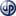# Generating test data with matlab and excel

Generating test data is not always about producing some random numbers. Sometimes it is about generating all possible combinations of given sets of attribute values. The following video shows how. Related matlab and excel VBA scripts for generating value combinations of independent and related attributes can be downloaded at download page.

The video above is about generating all possible value combinations of independent attributes.Because these attributes are not inter-related, all possible combinations are valid, as shown in the figure at the left. Accordingly, if we have three independent attributes like Car, Color and Type, each with 3, 4 and 2 values respectively, we will have a total of 24 (3 x 4 x 2) distinct attribute combinations.

What if we want to generate all possible combinations of hierarchically related attributes like Origin, Car and Model as shown in the figure below?Hierarchical relation means, there is 1-N relation between parent (higher) and child (lower) attributes. For example, a Car brand (child) can have only one Origin (parent), but an Origin can be shared by multiple Cars. Due to this strict 1-N relation between parent and child attributes, there can be only one single path from a leaf (lowest) node to a root (topmost) node in the hierarchical three structure. That is, the total number of possible paths from root to leaf nodes is equal to the total number of leaf nodes. Note that each possible path from root to leaf represents a possible attribute value combination.The whole hierarchy information can be represented with attribute value pairs as shown in the figure on the left. Once the pairwise attribute relations are formulated with such vector pairs, all possible combinations of attribute values (which is the complete hierarchy table) can easily be generated. The trick in the generation algorithm is excluding invalid value combinations as shown in the figure. You can find information about such algorithmic details in supplementary PowerPoint slides.

As already mentioned at the beginning, matlab and excel VBA scripts (makros) for generating test data can be downloaded at the central download page along with supplementary PowerPoint slides.

Tunç Ali Kütükçüoglu, 18. July 2012Copyright secured by Digiprove © 2012 Tunc Ali Kütükcüoglu
This entry was posted in Uncategorized and tagged , , , , . Bookmark the permalink.